/scikit-learn

# sklearn.linear_model.LinearRegression

`class sklearn.linear_model.LinearRegression(fit_intercept=True, normalize=False, copy_X=True, n_jobs=None)` [source]

Ordinary least squares Linear Regression.

Parameters: `fit_intercept : boolean, optional, default True` whether to calculate the intercept for this model. If set to False, no intercept will be used in calculations (e.g. data is expected to be already centered). `normalize : boolean, optional, default False` This parameter is ignored when `fit_intercept` is set to False. If True, the regressors X will be normalized before regression by subtracting the mean and dividing by the l2-norm. If you wish to standardize, please use `sklearn.preprocessing.StandardScaler` before calling `fit` on an estimator with `normalize=False`. `copy_X : boolean, optional, default True` If True, X will be copied; else, it may be overwritten. `n_jobs : int or None, optional (default=None)` The number of jobs to use for the computation. This will only provide speedup for n_targets > 1 and sufficient large problems. `None` means 1 unless in a `joblib.parallel_backend` context. `-1` means using all processors. See Glossary for more details. `coef_ : array, shape (n_features, ) or (n_targets, n_features)` Estimated coefficients for the linear regression problem. If multiple targets are passed during the fit (y 2D), this is a 2D array of shape (n_targets, n_features), while if only one target is passed, this is a 1D array of length n_features. `intercept_ : array` Independent term in the linear model.

#### Notes

From the implementation point of view, this is just plain Ordinary Least Squares (scipy.linalg.lstsq) wrapped as a predictor object.

#### Examples

```>>> import numpy as np
>>> from sklearn.linear_model import LinearRegression
>>> X = np.array([[1, 1], [1, 2], [2, 2], [2, 3]])
>>> # y = 1 * x_0 + 2 * x_1 + 3
>>> y = np.dot(X, np.array([1, 2])) + 3
>>> reg = LinearRegression().fit(X, y)
>>> reg.score(X, y)
1.0
>>> reg.coef_
array([1., 2.])
>>> reg.intercept_
3.0000...
>>> reg.predict(np.array([[3, 5]]))
array([16.])
```

#### Methods

 `fit`(X, y[, sample_weight]) Fit linear model. `get_params`([deep]) Get parameters for this estimator. `predict`(X) Predict using the linear model `score`(X, y[, sample_weight]) Returns the coefficient of determination R^2 of the prediction. `set_params`(**params) Set the parameters of this estimator.
`__init__(fit_intercept=True, normalize=False, copy_X=True, n_jobs=None)` [source]
`fit(X, y, sample_weight=None)` [source]

Fit linear model.

Parameters: `X : array-like or sparse matrix, shape (n_samples, n_features)` Training data `y : array_like, shape (n_samples, n_targets)` Target values. Will be cast to X’s dtype if necessary `sample_weight : numpy array of shape [n_samples]` Individual weights for each sample New in version 0.17: parameter sample_weight support to LinearRegression. `self : returns an instance of self.`
`get_params(deep=True)` [source]

Get parameters for this estimator.

Parameters: `deep : boolean, optional` If True, will return the parameters for this estimator and contained subobjects that are estimators. `params : mapping of string to any` Parameter names mapped to their values.
`predict(X)` [source]

Predict using the linear model

Parameters: `X : array_like or sparse matrix, shape (n_samples, n_features)` Samples. `C : array, shape (n_samples,)` Returns predicted values.
`score(X, y, sample_weight=None)` [source]

Returns the coefficient of determination R^2 of the prediction.

The coefficient R^2 is defined as (1 - u/v), where u is the residual sum of squares ((y_true - y_pred) ** 2).sum() and v is the total sum of squares ((y_true - y_true.mean()) ** 2).sum(). The best possible score is 1.0 and it can be negative (because the model can be arbitrarily worse). A constant model that always predicts the expected value of y, disregarding the input features, would get a R^2 score of 0.0.

Parameters: `X : array-like, shape = (n_samples, n_features)` Test samples. For some estimators this may be a precomputed kernel matrix instead, shape = (n_samples, n_samples_fitted], where n_samples_fitted is the number of samples used in the fitting for the estimator. `y : array-like, shape = (n_samples) or (n_samples, n_outputs)` True values for X. `sample_weight : array-like, shape = [n_samples], optional` Sample weights. `score : float` R^2 of self.predict(X) wrt. y.
`set_params(**params)` [source]

Set the parameters of this estimator.

The method works on simple estimators as well as on nested objects (such as pipelines). The latter have parameters of the form `<component>__<parameter>` so that it’s possible to update each component of a nested object.

Returns: self

## Examples using `sklearn.linear_model.LinearRegression`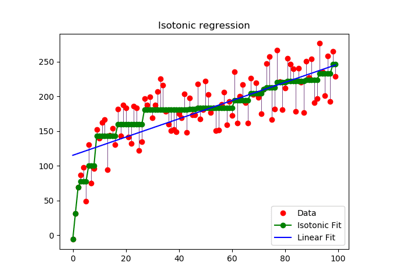Isotonic Regression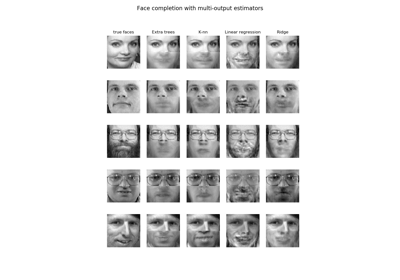Face completion with a multi-output estimators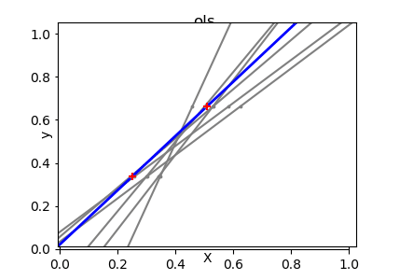Ordinary Least Squares and Ridge Regression Variance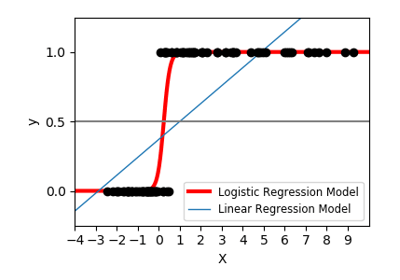Logistic function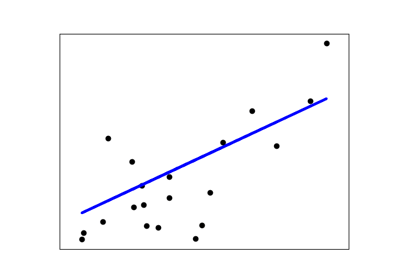Linear Regression Example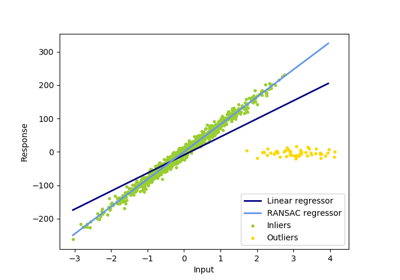Robust linear model estimation using RANSAC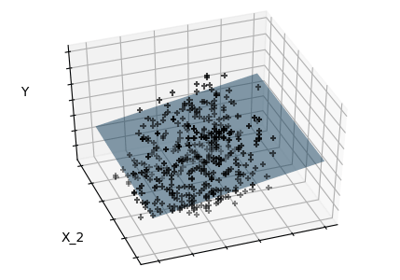Sparsity Example: Fitting only features 1 and 2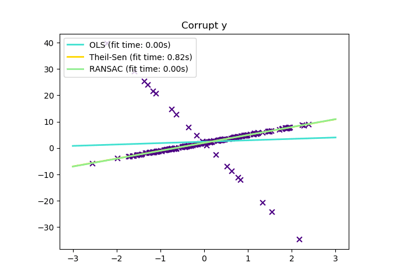Theil-Sen Regression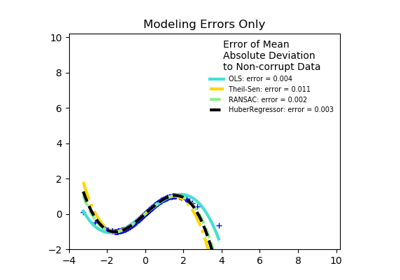Robust linear estimator fitting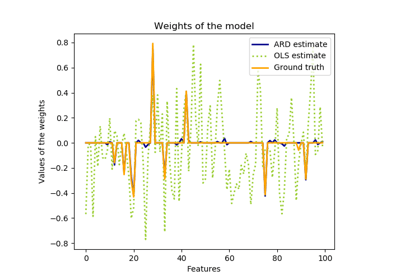Automatic Relevance Determination Regression (ARD)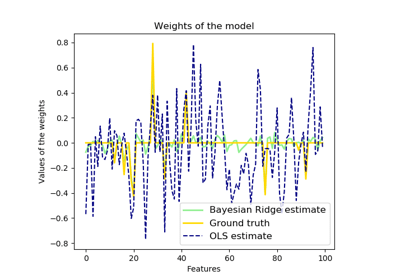Bayesian Ridge Regression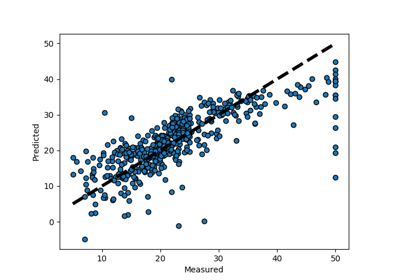Plotting Cross-Validated Predictions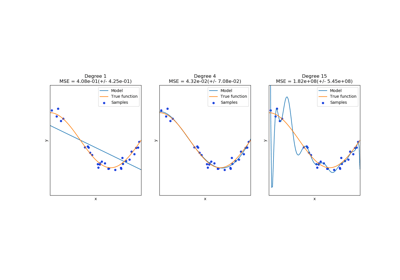Underfitting vs. Overfitting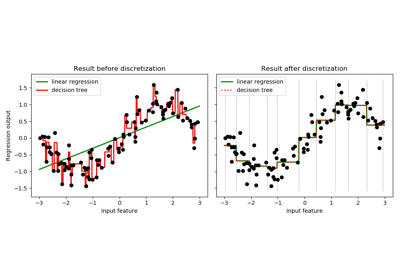Using KBinsDiscretizer to discretize continuous features